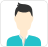true

Find the best tutors and institutes for Class 7 Tuition

Find Best Class 7 Tuition

No matching category found.

No matching Locality found.

Outside India?

Search in

# Learn Exercise 7.2 with Free Lessons & Tips

Which congruence criterion do you use in the following?

(a) Given: AC = DF

AB = DE

BC = EF

So, ΔABC ≅ ΔDEF(b) Given: ZX = RP

RQ = ZY

∠PRQ = ∠XZY

So, ΔPQR ≅ ΔXYZ(c) Given: ∠MLN = ∠FGH

∠NML = ∠GFH

ML = FG

So, ΔLMN ≅ ΔGFH(d) Given: EB = DB

AE = BC

∠A = ∠C = 90°

So, ΔABE ≅ ΔCDB(a) SSS, as the sides of ΔABC are equal to the sides of ΔDEF.

(b) SAS, as two sides and the angle included between these sides of ΔPQR are equal to two sides and the angle included between these sides of ΔXYZ.

(c) ASA, as two angles and the side included between these angles of ΔLMN are equal to two angles and the side included between these angles of ΔGFH.

(d) RHS, as in the given two right-angled triangles, one side and the hypotenuse are respectively equal.Harshit Bhardwaj

You want to show that ΔART ≅ ΔPEN,

(a) If you have to use SSS criterion, then you need to show

(i) AR = (ii) RT = (iii) AT =

(b) If it is given that ∠T = ∠N and you are to use SAS criterion, you need to have

(i) RT = and (ii) PN =

(c) If it is given that AT = PN and you are to use ASA criterion, you need to have

(i) ? (ii) ?(a)

(i) AR = PE

(ii) RT = EN

(iii) AT = PN

(b)

(i) RT = EN

(ii) PN = AT

(c)

(i) ∠ATR = ∠PNE

(ii) ∠RAT = ∠EPNHarshit Bhardwaj

You have to show that ΔAMP ≅ AMQ.

In the following proof, supply the missing reasons.

 - Steps - Reasons (i) PM = QM (i) … (ii) ∠PMA = ∠QMA (ii) … (iii) AM = AM (iii) … (iv) ΔAMP ≅ ΔAMQ (iv) …(i) Given

(ii) Given

(iii) Common

(iv) SAS, as the two sides and the angle included between these sides of ΔAMP are equal to two sides and the angle included between these sides of ΔAMQ.Harshit Bhardwaj

In ΔABC, ∠A = 30°, ∠B = 40° and ∠C = 110°

In ΔPQR, ∠P = 30°, ∠Q = 40° and ∠R = 110°

A student says that ΔABC ≅ ΔPQR by AAA congruence criterion. Is he justified? Why or why not?

No. This property represents that these triangles have their respective angles of equal measure. However, this gives no information about their sides. The sides of these triangles have a ratio somewhat different than 1:1. Therefore, AAA property does not prove the two triangles congruent.Harshit Bhardwaj

In the figure, the two triangles are congruent.

The corresponding parts are marked. We can write ΔRAT ≅ ?It can be observed that,

∠RAT = ∠WON

∠ART = ∠OWN

AR = OW

Therefore, ΔRATΔWON, by ASA criterion.Harshit Bhardwaj

Complete the congruence statement:

ΔBCA ≅?

ΔQRS ≅?Given that, BC = BT

TA = CA

BA is common.

Therefore, ΔBCAΔBTA

Similarly, PQ = RS

TQ = QS
PT = RQ

Therefore, ΔQRSΔTPQHarshit Bhardwaj

In a squared sheet, draw two triangles of equal areas such that

(i) The triangles are congruent.

(ii) The triangles are not congruent.

What can you say about their perimeters?

(i)Here, ΔABC and ΔPQR have the same area and are congruent to each other also. Also, the perimeter of both the triangles will be the same.

(ii)Here, the two triangles have the same height and base. Thus, their areas are equal. However, these triangles are not congruent to each other. Also, the perimeter of both the triangles will not be the same.Harshit Bhardwaj

If ΔABC and ΔPQR are to be congruent, name one additional pair of corresponding parts. What criterion did you use?BC = QR

ΔABCΔPQR (ASA criterion)Harshit Bhardwaj

Explain, why

ΔABC ≅ ΔFEDGiven that, ∠ABC = ∠FED (1)

∠BAC = ∠EFD (2)

The two angles of ΔABC are equal to the two respective angles of ΔFED. Also, the sum of all interior angles of a triangle is 180º. Therefore, third angle of both triangles will also be equal in measure.

∠BCA = ∠EDF (3)

Also, given that, BC = ED (4)

By using equation (1), (3), and (4), we obtain

ΔABC ≅ ΔFED (ASA criterion)Harshit Bhardwaj

Explain, why

ΔABC ≅ ΔFEDGiven that, ∠ABC = ∠FED (1)

∠BAC = ∠EFD (2)

The two angles of ΔABC are equal to the two respective angles of ΔFED. Also, the sum of all interior angles of a triangle is 180º. Therefore, third angle of both triangles will also be equal in measure.

∠BCA = ∠EDF (3)

Also, given that, BC = ED (4)

By using equation (1), (3), and (4), we obtain

ΔABC ≅ ΔFED (ASA criterion)Tasneem

Draw a rough sketch of two triangles such
that they have five pairs of congruent parts
but still the triangles are not congruent?

A pair of triangles with three equal angles, and two equal sides are non-congruent.

Ex:Charlie

How can we Improve it?

Please enter your question below and we will send it to our tutor communities to answer it *

Name *

Enter a valid name.

Email *

Enter a valid email.

Email or Mobile Number: *

UrbanPro.com helps you to connect with the best Class 7 Tuition in India. Post Your Requirement today and get connected.

X

### Looking for Class 7 Tuition Classes?

Find best tutors for Class 7 Tuition Classes by posting a requirement.

• Post a learning requirement
• Get customized responses
• Compare and select the best### Looking for Class 7 Tuition Classes?

Find best Class 7 Tuition Classes in your locality on UrbanPro